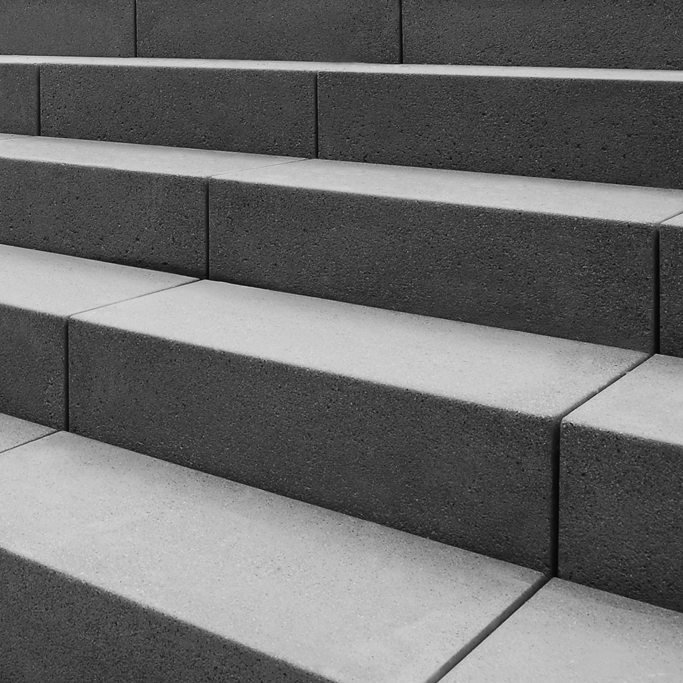# StepsA small terrace at a flat football ground has 15 steps, each of which is 50 m long and built of solid concrete. Each step has a rise of $\frac{1}{4}$ m and a tread of $\frac{1}{2}$ m.Calculate the total volume of concrete required to built the terrace.

Note: You cannot build a step in midair. You have to fill the air beneath it.

×